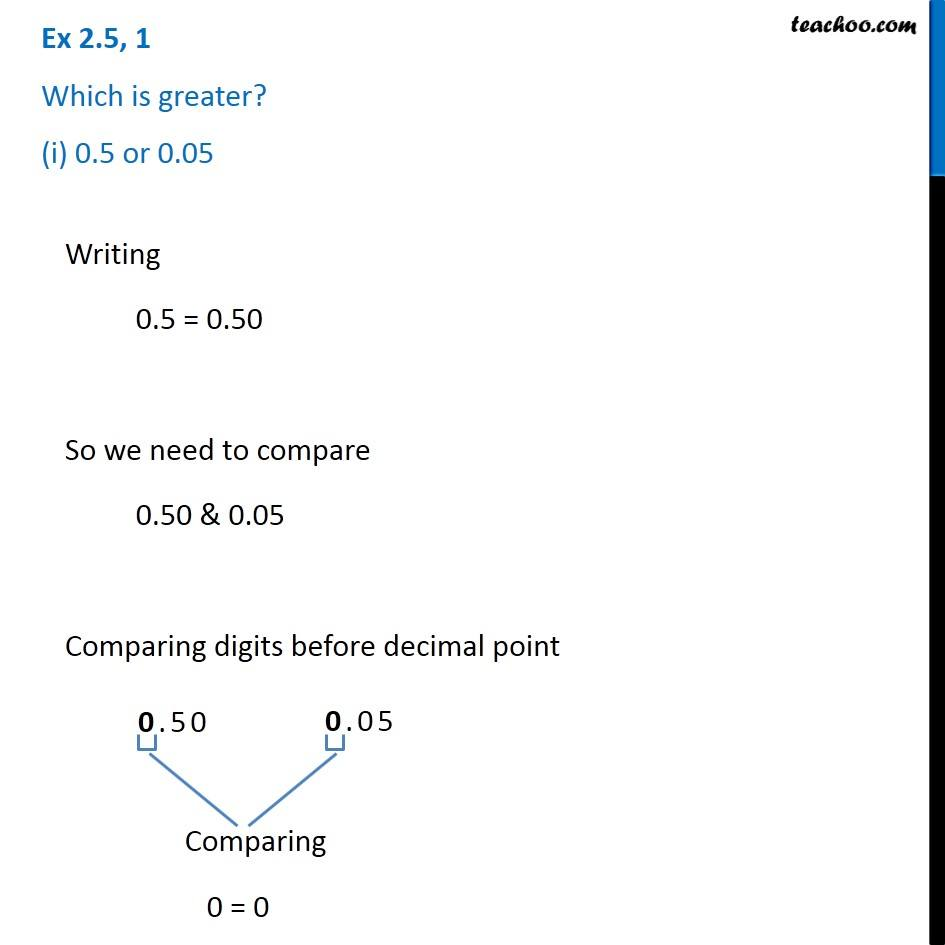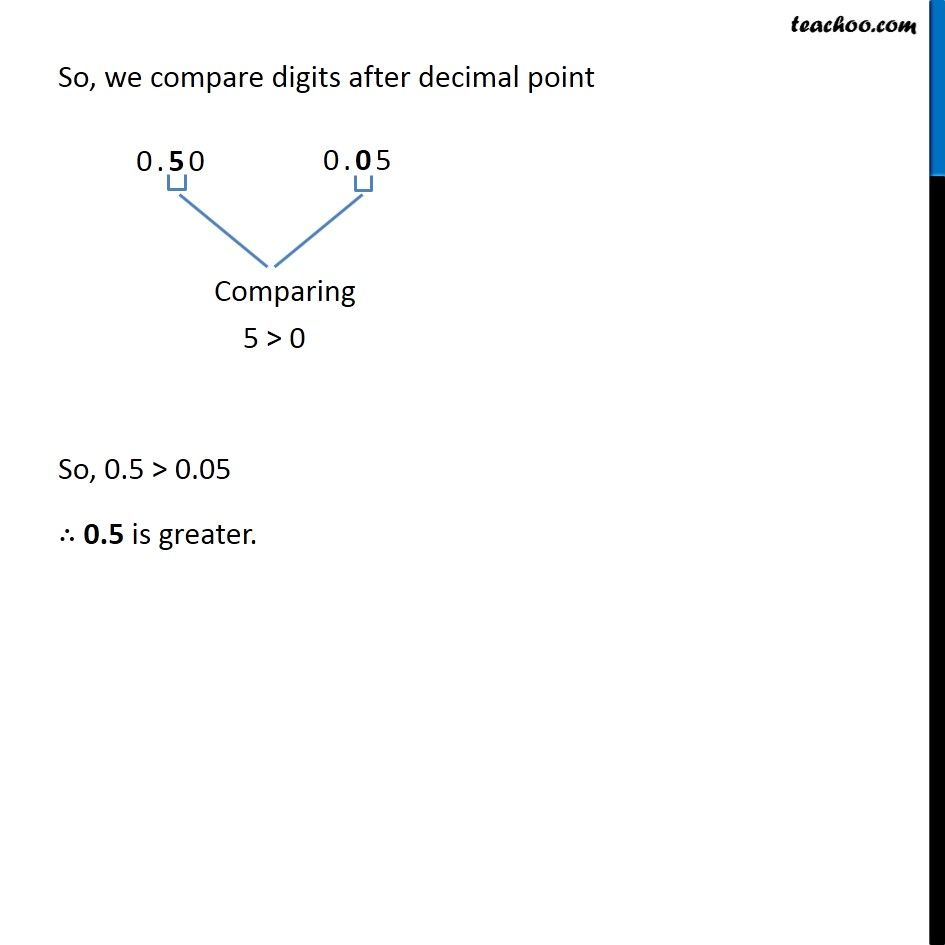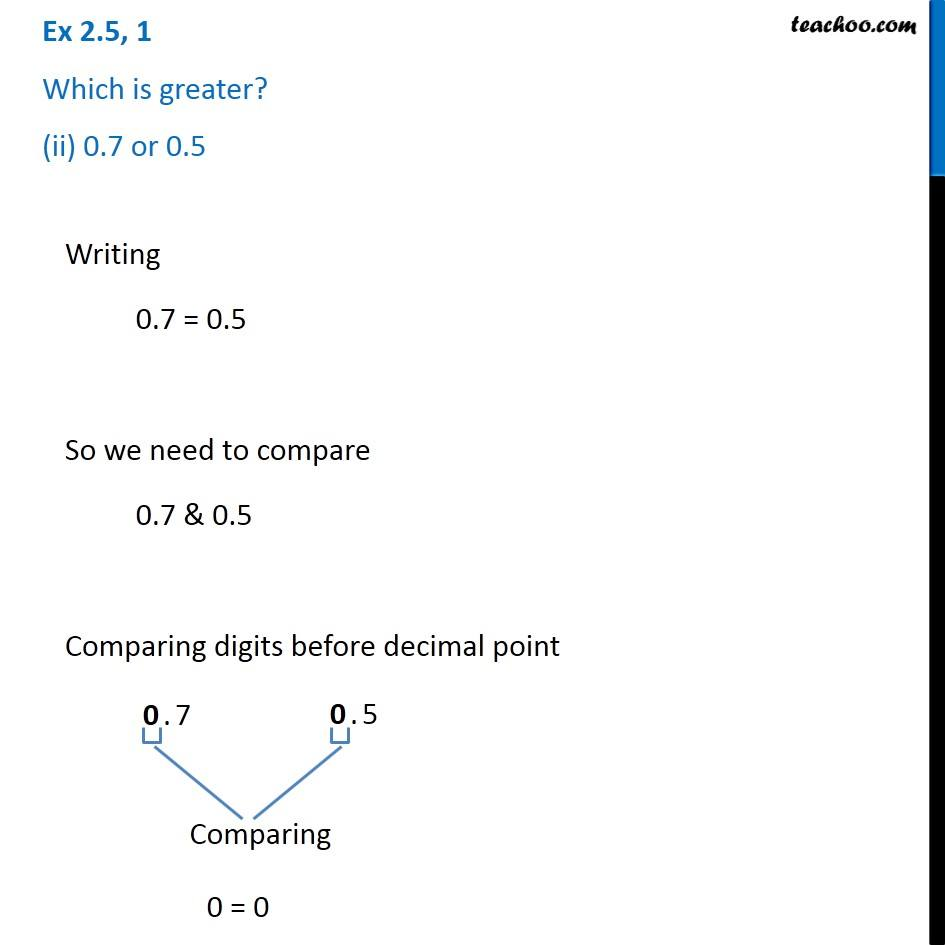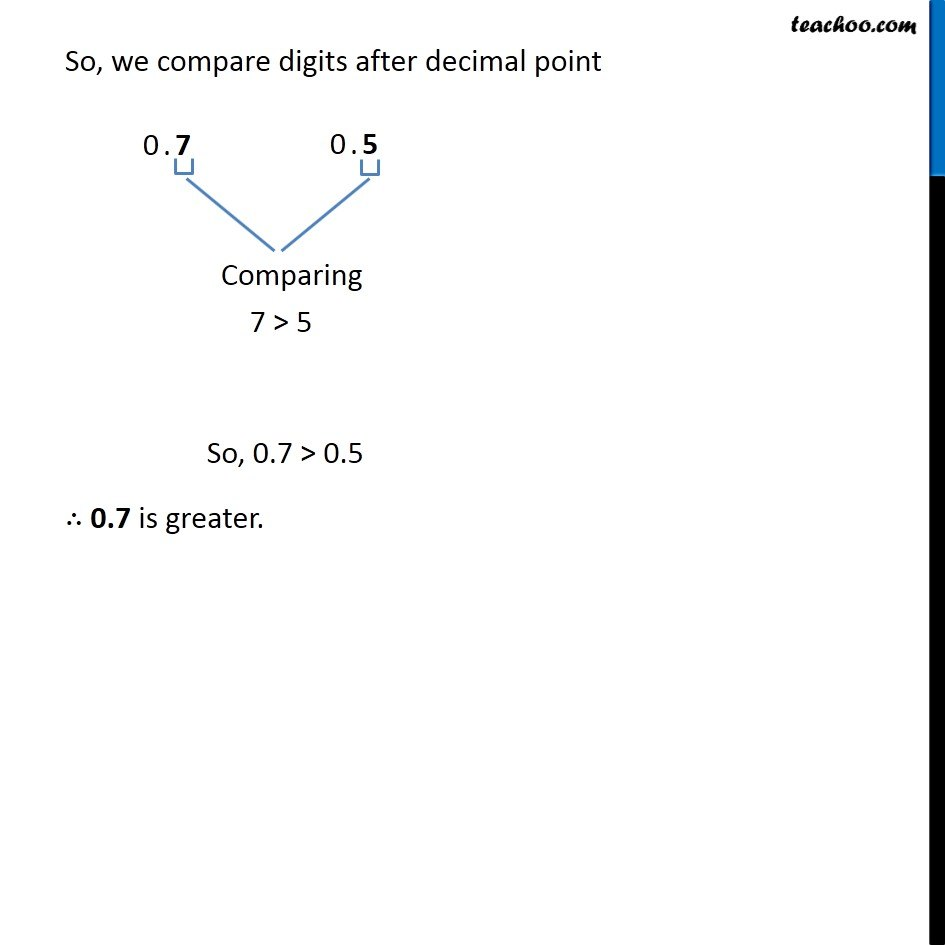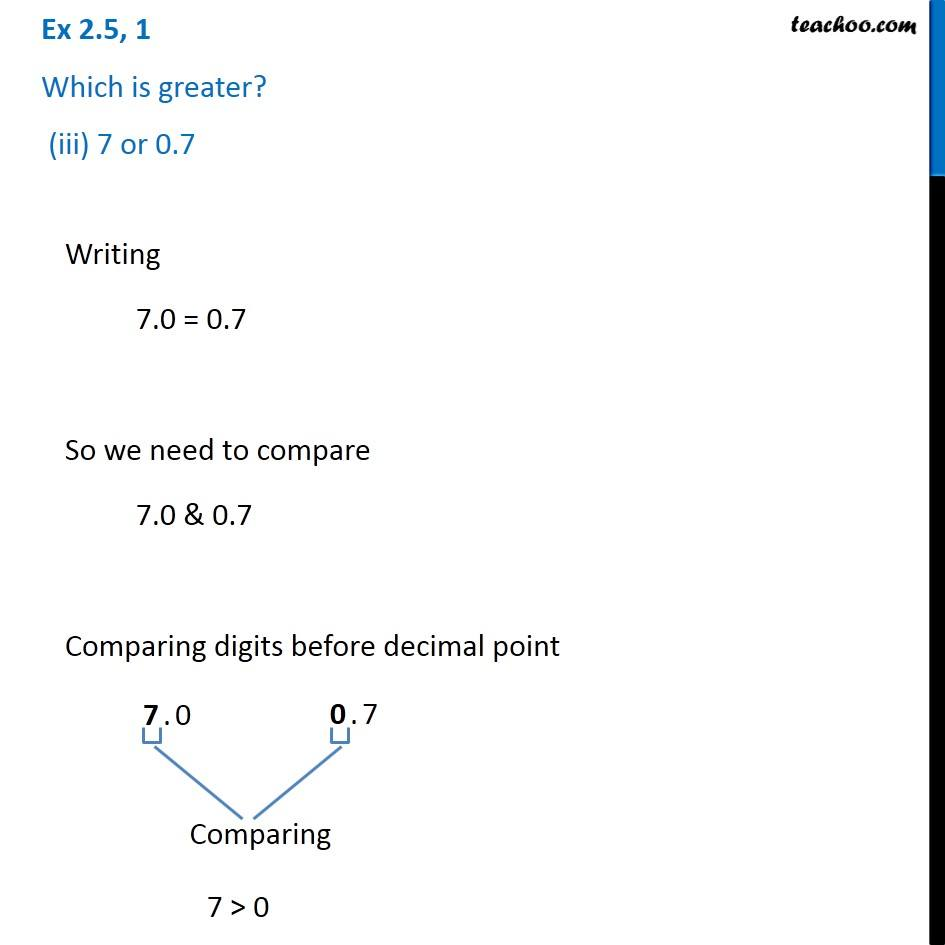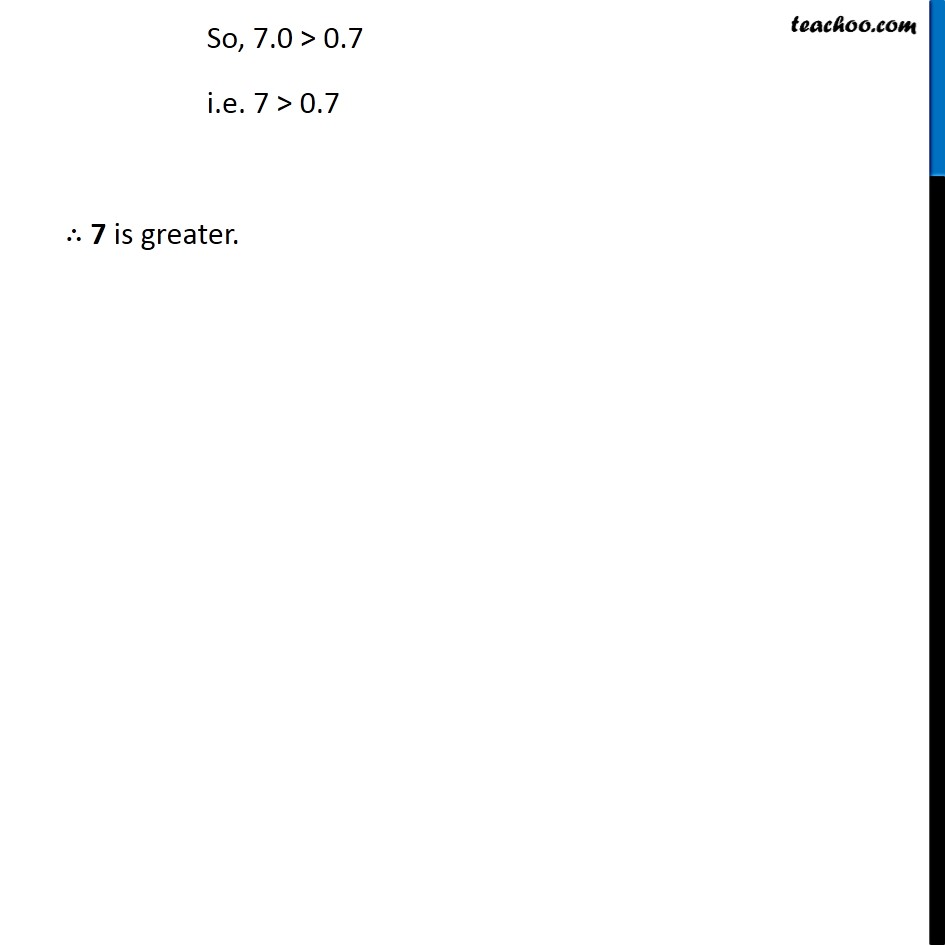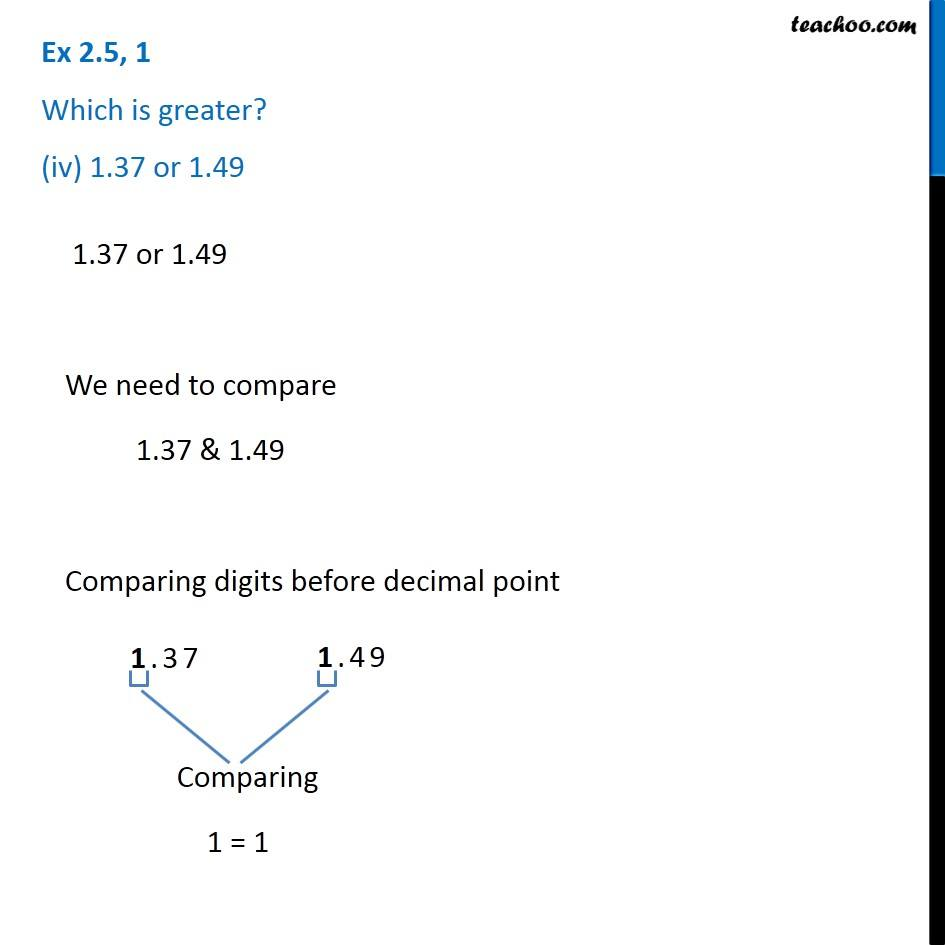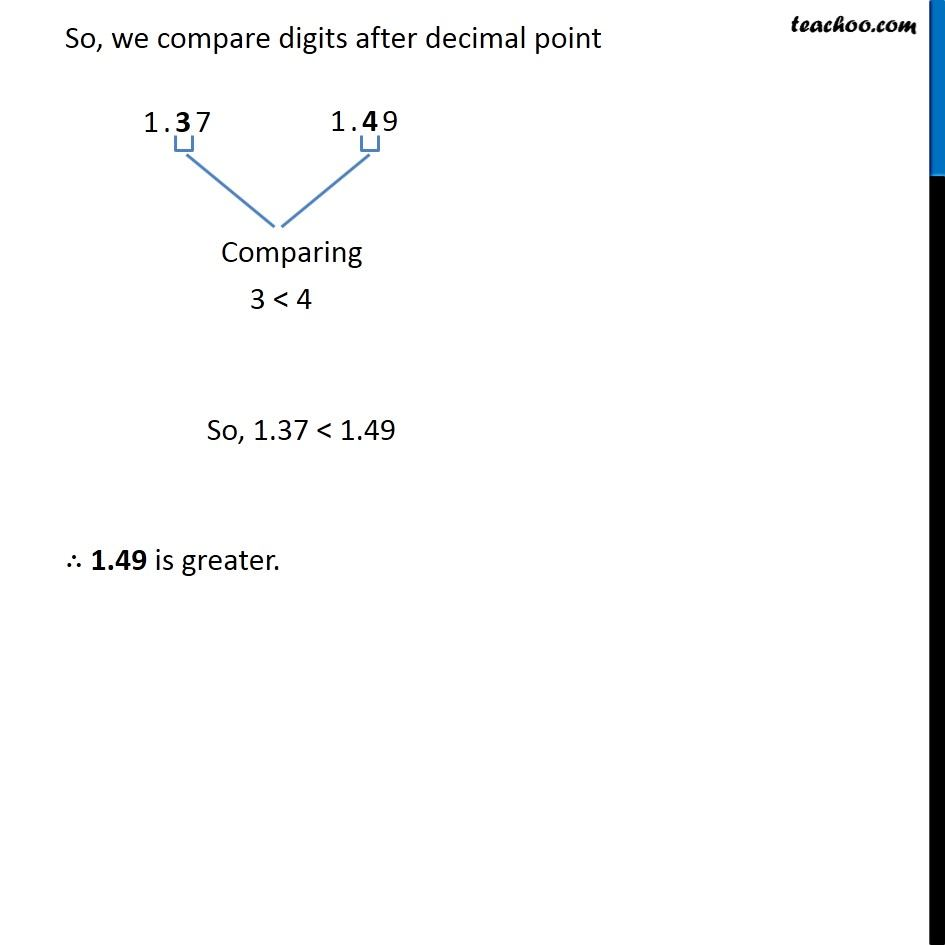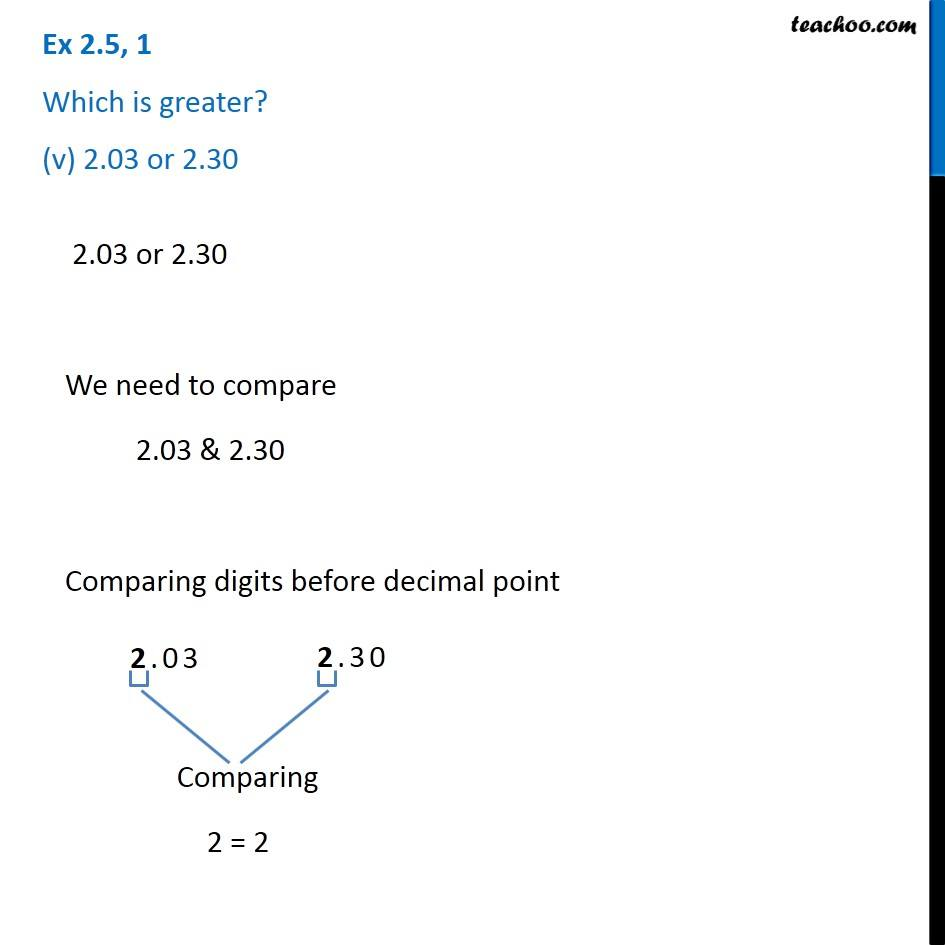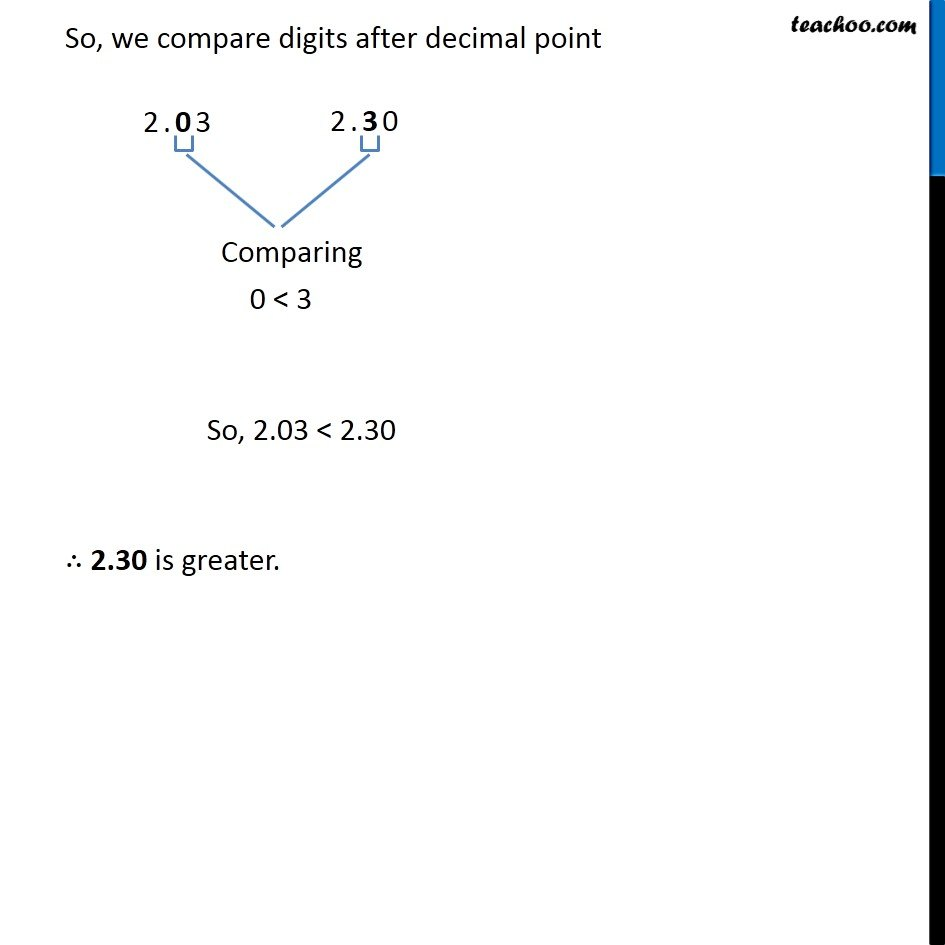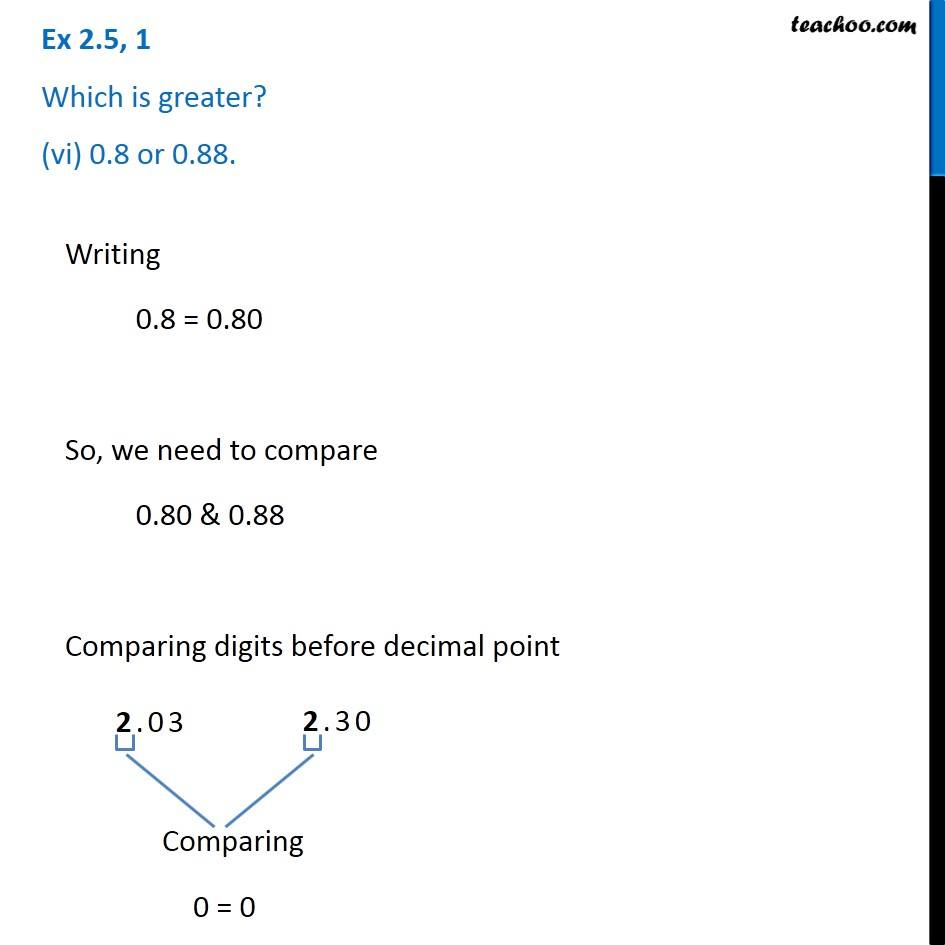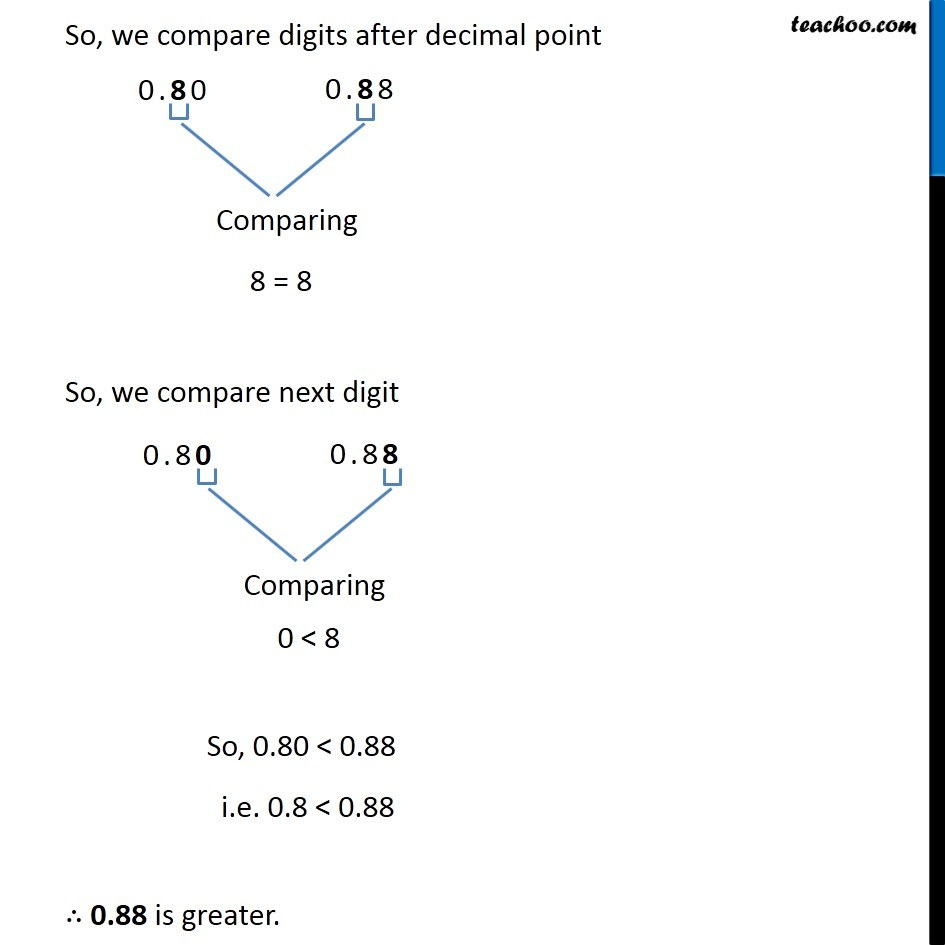Subscribe to our Youtube Channel - https://you.tube/teachoo

1. Chapter 2 Class 7 Fractions and Decimals
2. Serial order wise
3. Ex 2.5

Transcript

Ex 2.5, 1 Which is greater? (i) 0.5 or 0.05 Writing 0.5 = 0.50 So we need to compare 0.50 & 0.05 Comparing digits before decimal point 0 = 0 So, we compare digits after decimal point 5 > 0 So, 0.5 > 0.05 ∴ 0.5 is greater. So, we compare digits after decimal point 5 > 0 So, 0.5 > 0.05 ∴ 0.5 is greater. Ex 2.5, 1 Which is greater? (ii) 0.7 or 0.5 Writing 0.7 = 0.5 So we need to compare 0.7 & 0.5 Comparing digits before decimal point 0 = 0 So, we compare digits after decimal point 7 > 5 So, 0.7 > 0.5 ∴ 0.7 is greater. Ex 2.5, 1 Which is greater? (iii) 7 or 0.7 Writing 7.0 = 0.7 So we need to compare 7.0 & 0.7 Comparing digits before decimal point 7 > 0 So, 7.0 > 0.7 i.e. 7 > 0.7 ∴ 7 is greater. Ex 2.5, 1 Which is greater? (iv) 1.37 or 1.49 1.37 or 1.49 We need to compare 1.37 & 1.49 Comparing digits before decimal point 1 = 1 So, we compare digits after decimal point 3 < 4 So, 1.37 < 1.49 ∴ 1.49 is greater. Ex 2.5, 1 Which is greater? (v) 2.03 or 2.30 2.03 or 2.30 We need to compare 2.03 & 2.30 Comparing digits before decimal point 2 = 2 So, we compare digits after decimal point 0 < 3 So, 2.03 < 2.30 ∴ 2.30 is greater. Ex 2.5, 1 Which is greater? (vi) 0.8 or 0.88. Writing 0.8 = 0.80 So, we need to compare 0.80 & 0.88 Comparing digits before decimal point 0 = 0 So, we compare digits after decimal point 8 = 8 So, we compare next digit 0 < 8 So, 0.80 < 0.88 i.e. 0.8 < 0.88 ∴ 0.88 is greater.

Ex 2.5